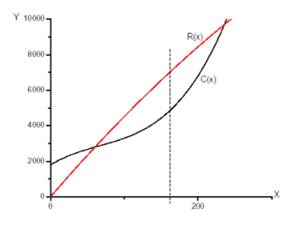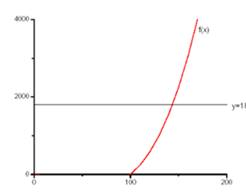# The production level for maximum profit by the graphs .### Single Variable Calculus: Concepts...

4th Edition
James Stewart
Publisher: Cengage Learning
ISBN: 9781337687805### Single Variable Calculus: Concepts...

4th Edition
James Stewart
Publisher: Cengage Learning
ISBN: 9781337687805

#### Solutions

Chapter 4, Problem 48RE

(a)

To determine

## To find: The production level for maximum profit by the graphs.

Expert Solution

The production level of x=160 units will be maximum profit

### Explanation of Solution

Given information:

The given information is

Commodity is C(x)=1800+25x0.2x2+0.001x3

Demand function is p(x)=48.20.03x .

Calculation:

The cost function is C(x)=1800+25x0.2x2+0.001x3

Demand function is p(x)=48.20.03x

The revenue function R(x)=x.p(x)

Or R(x)=48.2x0.03x2

Now we draw the curve of C(x) and R(x) on the same screen and get that Manufacturers has profit when R>C , and maximum profit occurs when R and C have parallel tangents.

That is at the production level of x=160 units.Therefore, the production level of x=160 units will be maximum profit.

(b)

To determine

### To find: The production level for maximum profit by calculus method.

Expert Solution

The production level 161 units will maximize the profit.

### Explanation of Solution

Given information:

The given information is

Commodity is C(x)=1800+25x0.2x2+0.001x3

Demand function is p(x)=48.20.03x .

Calculation:

Now the marginal revenue function is

R'(x)=48.20.06x

And marginal cost function is

C'(x)=25x0.4x+0.003x3

For maximize the profit we must have

R'(x)=C'(x)

Or 48.20.06x=25x0.4x+0.003x30.003x20.34x23.2=0

The root of the equation ax2+bx+c=0

x=b±b24ac2a

Here we are not considering x=bb24ac2a because x>0 .

Thus we have

x=0.34+(0.34)24×0.003×23.20.006

x=161 Units.

Since R'(x)= 0.06 and C'(x)= 0.006x0.4

So R'(x)<C(x) for x=161 units

Therefore, the production level 161 units will maximize the profit.

(c)

To determine

### To find: The production level that minimizes the average cost.

Expert Solution

The production level of 144 units the average cost is minimum

### Explanation of Solution

Given information:

The given information is

Commodity is C(x)=1800+25x0.2x2+0.001x3

Demand function is p(x)=48.20.03x .

Calculation:

Now the average cost function is

c(x)=C(x)x=1800x+250.2x+0.001x2

And marginal cost is

C'(x)=25x0.4x+0.003x3

For minimum average cost we must have

c(x)=C'(x)

1800x+250.2x+0.001x2=25x0.4x+0.003x30.002x30.2x2=1800

By graphical method

We draw the curve f(x)=0.002x30.2x2 and y=1800 .We estimate the point of intersection of these curves. The x-coordinate of the point of intersection is about 144 units.

So at the production level of 144 units the average cost is minimum.

Now,

c(x)=C(x)x=1800x+250.2x+0.001x2

Then c'(x)=1800x20.2+0.002x

And c''(x)=2×1800x3+0.002>0 for all x>0

So at x=144,c(x) has an absolute minimum.

### Have a homework question?

Subscribe to bartleby learn! Ask subject matter experts 30 homework questions each month. Plus, you’ll have access to millions of step-by-step textbook answers!